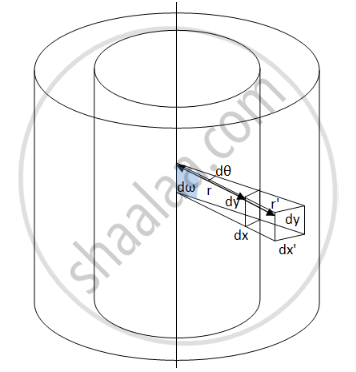Department of Pre-University Education, KarnatakaPUC Karnataka Science Class 12

# The Intensity Produced by a Long Cylindrical Light Source at a Small Distance R from the Source is Proportional to - Physics

MCQ
Fill in the Blanks

The intensity produced by a long cylindrical light source at a small distance r from the source is proportional to _________ .

#### Options

• $\frac{1}{r^2}$

• $\frac{1}{r^3}$

• $\frac{1}{r}$

• None of these

#### Solution

$\frac{1}{r}$Let us consider two coaxial cylindrical surfaces at distances r and r' from the axis. Let areas dA and dA' subtend the solid angle d​ω at the central axis. The height of the area element will be same, i.e. equal to dy.  Let the breath of dA be dx and that of dA' be dx'.

Now from the arcs,

$dx = rd\theta$

$dx' = r'd\theta$

Now,

$dA = dxdy = rd\theta\ dy$

$dA' = dx'dy = r'd\theta\ dy$

$\frac{dA}{dA'} = \frac{r}{r'}$

$\Rightarrow \frac{dA}{r} = \frac{dA'}{r'} = d\omega$

The luminous flux going through the solid angle d​ω will be

dF = I​dω

Now,

$dF = I\frac{dA}{r}$

If the surfaces are inclined at an angle $\alpha,$

$dF = I\frac{dA\cos\alpha}{r}$

Now, illuminance is defined as

$E = \frac{dF}{dA} = I\frac{dA\cos\alpha}{r}$

$\Rightarrow E \propto \frac{1}{r}$

Concept: Light Process and Photometry
Is there an error in this question or solution?

#### APPEARS IN

HC Verma Class 11, Class 12 Concepts of Physics Vol. 1
Chapter 22 Photometry
MCQ | Q 7 | Page 454
Share